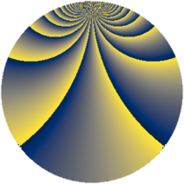# Properties

 Label 507.2.fLevel $507$ Weight $2$ Character orbit 507.f Rep. character $\chi_{507}(239,\cdot)$ Character field $\Q(\zeta_{4})$ Dimension $84$ Newform subspaces $7$ Sturm bound $121$ Trace bound $7$

# Related objects

## Defining parameters

 Level: $$N$$ $$=$$ $$507 = 3 \cdot 13^{2}$$ Weight: $$k$$ $$=$$ $$2$$ Character orbit: $$[\chi]$$ $$=$$ 507.f (of order $$4$$ and degree $$2$$) Character conductor: $$\operatorname{cond}(\chi)$$ $$=$$ $$39$$ Character field: $$\Q(i)$$ Newform subspaces: $$7$$ Sturm bound: $$121$$ Trace bound: $$7$$ Distinguishing $$T_p$$: $$2$$, $$5$$, $$7$$

## Dimensions

The following table gives the dimensions of various subspaces of $$M_{2}(507, [\chi])$$.

Total New Old
Modular forms 148 124 24
Cusp forms 92 84 8
Eisenstein series 56 40 16

## Trace form

 $$84 q + 4 q^{3} + 4 q^{6} - 4 q^{7} + 4 q^{9} + O(q^{10})$$ $$84 q + 4 q^{3} + 4 q^{6} - 4 q^{7} + 4 q^{9} - 8 q^{15} - 12 q^{16} - 8 q^{18} - 4 q^{19} + 4 q^{21} + 24 q^{22} - 12 q^{24} - 56 q^{27} - 4 q^{28} + 20 q^{31} + 16 q^{33} - 4 q^{37} - 16 q^{40} + 16 q^{45} - 24 q^{46} - 88 q^{48} + 4 q^{54} - 40 q^{55} + 4 q^{57} - 8 q^{58} + 8 q^{60} - 24 q^{61} + 4 q^{63} - 36 q^{66} + 20 q^{67} - 8 q^{70} + 24 q^{72} - 4 q^{73} + 4 q^{76} - 8 q^{79} + 28 q^{81} + 4 q^{84} + 40 q^{87} - 20 q^{93} + 64 q^{94} - 20 q^{96} - 28 q^{97} - 32 q^{99} + O(q^{100})$$

## Decomposition of $$S_{2}^{\mathrm{new}}(507, [\chi])$$ into newform subspaces

Label Dim $A$ Field CM Traces $q$-expansion
$a_{2}$ $a_{3}$ $a_{5}$ $a_{7}$
507.2.f.a $4$ $4.048$ $$\Q(\zeta_{8})$$ None $$0$$ $$-4$$ $$0$$ $$-4$$ $$q+\zeta_{8}q^{2}+(-1-\zeta_{8}-\zeta_{8}^{3})q^{3}-\zeta_{8}^{2}q^{4}+\cdots$$
507.2.f.b $4$ $4.048$ $$\Q(\zeta_{12})$$ $$\Q(\sqrt{-3})$$ $$0$$ $$0$$ $$0$$ $$-2$$ $$q+(\zeta_{12}-\zeta_{12}^{2}+\zeta_{12}^{3})q^{3}+2\zeta_{12}^{2}q^{4}+\cdots$$
507.2.f.c $4$ $4.048$ $$\Q(\zeta_{12})$$ $$\Q(\sqrt{-3})$$ $$0$$ $$0$$ $$0$$ $$2$$ $$q+(\zeta_{12}-\zeta_{12}^{2}+\zeta_{12}^{3})q^{3}+2\zeta_{12}^{2}q^{4}+\cdots$$
507.2.f.d $8$ $4.048$ 8.0.56070144.2 $$\Q(\sqrt{-39})$$ $$0$$ $$0$$ $$0$$ $$0$$ $$q-\beta _{1}q^{2}+\beta _{4}q^{3}+(-2\beta _{2}-\beta _{5})q^{4}+\cdots$$
507.2.f.e $8$ $4.048$ 8.0.56070144.2 None $$0$$ $$4$$ $$0$$ $$-8$$ $$q+(\beta _{1}+\beta _{2}+\beta _{4})q^{2}+(\beta _{4}+\beta _{5})q^{3}+\cdots$$
507.2.f.f $8$ $4.048$ 8.0.56070144.2 None $$0$$ $$4$$ $$0$$ $$8$$ $$q+(\beta _{1}+\beta _{2}+\beta _{4})q^{2}+(1-\beta _{2}-\beta _{4}+\cdots)q^{3}+\cdots$$
507.2.f.g $48$ $4.048$ None $$0$$ $$0$$ $$0$$ $$0$$

## Decomposition of $$S_{2}^{\mathrm{old}}(507, [\chi])$$ into lower level spaces

$$S_{2}^{\mathrm{old}}(507, [\chi]) \cong$$ $$S_{2}^{\mathrm{new}}(39, [\chi])$$$$^{\oplus 2}$$